Open in App
Not now

# Olympics Data Analysis Using Python

• Difficulty Level : Medium
• Last Updated : 13 May, 2022

In this article, we are going to see the Olympics analysis using Python. The modern Olympic Games or Olympics are leading international sports events featuring summer and winter sports competitions in which thousands of athletes from around the world participate in a variety of competitions. The Olympic Games are considered the world’s foremost sports competition with more than 200 nations participating.

The total number of events in the Olympics is 339 in 33 sports. And for every event there are winners. Therefore various data is generated. So, by using Python we will analyze this data.

## Modules Used

• Pandas: It is used for analyzing the data,
• NumPy: NumPy is a general-purpose array-processing package.
• Matplotlib: It is a numerical mathematics extension NumPy
• seaborn: It is used for visualization statistical graphics plotting in Python

## Model architecture :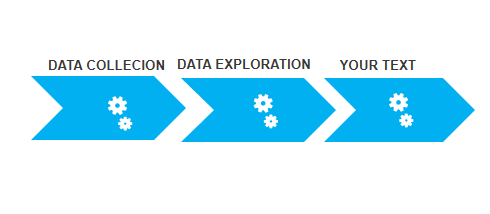## Python3

 `# importing all necessary libraries` `import` `pandas as pd` `import` `numpy as np` `import` `matplotlib.pyplot as plt` `import` `seaborn as sns`

### Step 2: Understanding database

When dealing with Olympic data, we have two CSV files. One containing outturn sports-related costs of the Olympic Games of all years. And other is containing the information about athletes of all years when they participated with information.

### Step 3: Data cleaning and formatting

We imported both the datasets using the .read_csv() method into a dataframe using pandas and displayed the first 5 rows of each dataset.

## Python3

 `data ``=` `pd.read_csv(``'athlete_events.csv'``)  ``# read file`   `# data.head() display first 5 entry` `# data.describe  about model` `# data.info give info about data` `print``(data.head(), data.describe(), data.info())`

Output: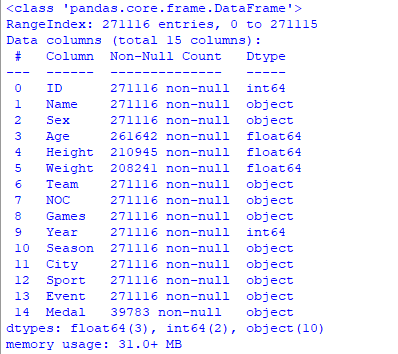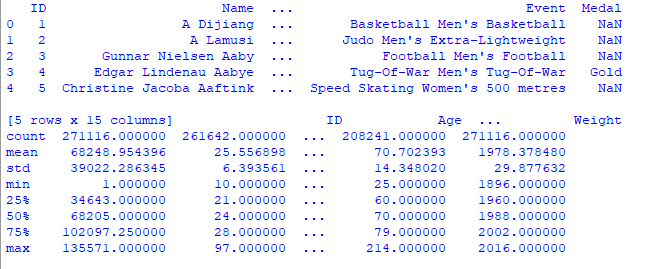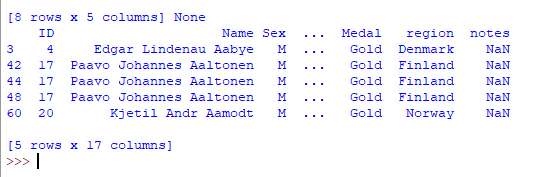### Step 4: Merging two DataFrame

Here we are going to merge two dataframe using pandas.merge() in python.

## Python3

 `# regions and country noc data csv file` `regions ``=` `pd.read_csv(``'datasets_31029_40943_noc_regions.csv'``)` `print``(regions.head())`   `# merging to data and regions frame` `merged ``=` `pd.merge(data, regions, on``=``'NOC'``, how``=``'left'``)` `print``(merged.head())`

Output: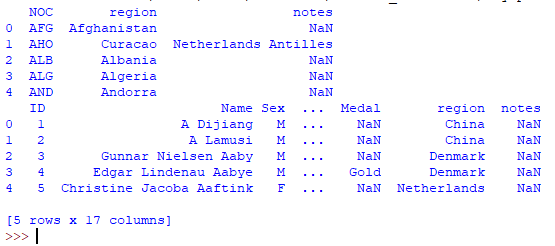## Data analysis of Olympics

Data is now available now using pandas and matplotlib lets see some examples

### Data analysis of Gold medalists

Creating a new data frame including only gold medalists.

## Python3

 `# creating goldmedal dataframes` `goldMedals ``=` `merged[(merged.Medal ``=``=` `'Gold'``)]` `print``(goldMedals.head())`

Output :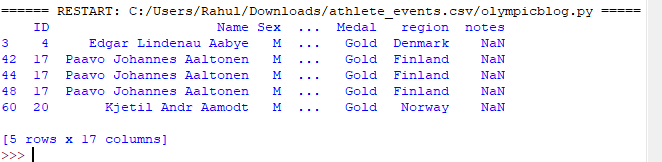### Gold medalist in respect of age:

Here we are going to create a graph of the number of gold medals with respect to age. For this, we will create countplot for graph representation which shows the X-axis as the age of the players and the Y-axis represent the number of medals.

## Python3

 `plt.figure(figsize``=``(``20``, ``10``))` `plt.title(``'Distribution of Gold Medals'``)` `sns.countplot(goldMedals[``'Age'``])` `plt.show()`

Output :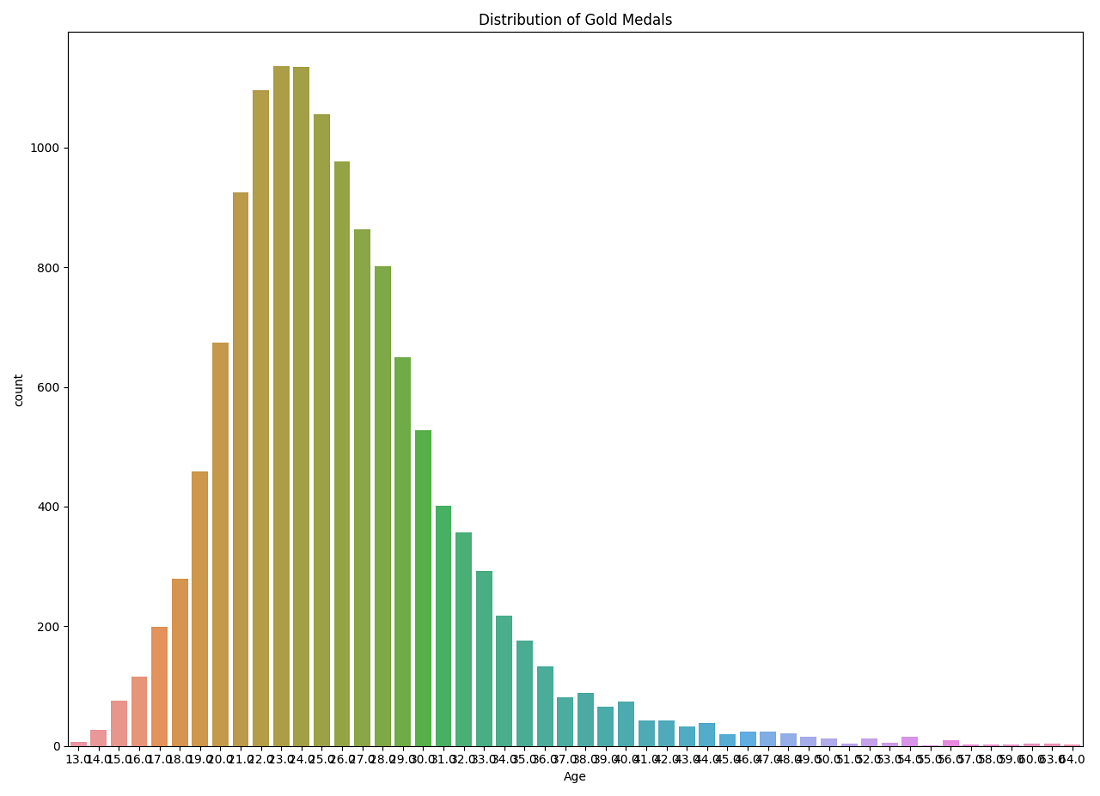Print the number of athletes who are gold medalists and whose age is greater than 50 with their info.

## Python3

 `goldMedals ``=` `merged[(merged.Medal ``=``=` `'Gold'``)]` `print``(``'The no of athletes is'``,` `      ``goldMedals[``'ID'``][goldMedals[``'Age'``] > ``50``].count(), ``'\n'``)` `print``(goldMedals[goldMedals[``'Age'``] > ``50``])`

Output :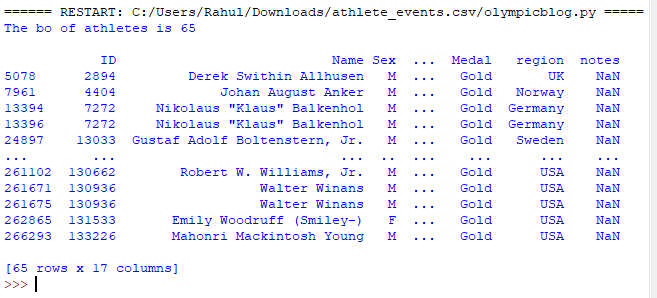Create a new dataframe called masterDisciplines in which we will insert this new set of people and then create a visualization with it

## Python3

 `masterDisciplines ``=` `goldMedals[``'Sport'``][goldMedals[``'Age'``] > ``50``]` `plt.figure(figsize``=``(``20``, ``10``))` `plt.tight_layout()` `sns.countplot(masterDisciplines)` `plt.title(``'Gold Medals for Athletes Over 50'``)` `plt.show()`

Output :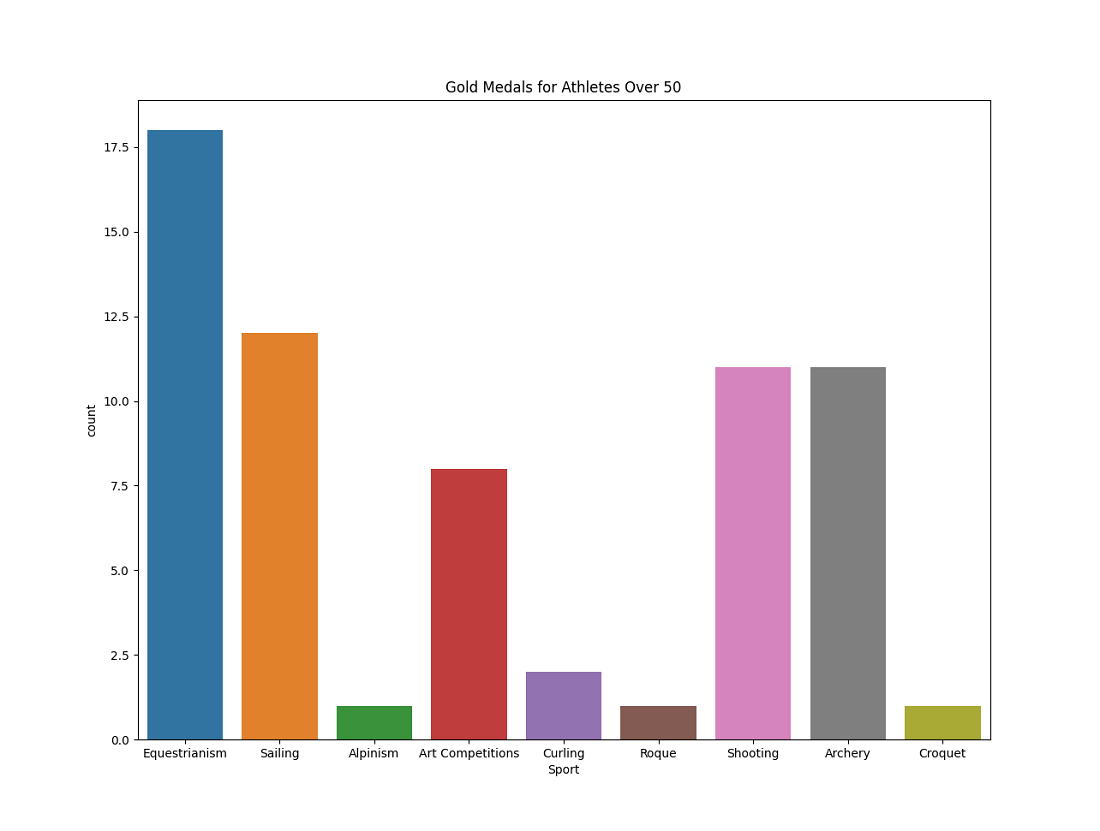### Women who can play in summer

Display all women athletes who have played in the summer season and it show the increase in women athletes after a long period via graphical representation.

## Python3

 `womenInOlympics ``=` `merged[(merged.Sex ``=``=` `'F'``) &` `                         ``(merged.Season ``=``=` `'Summer'``)]` `print``(womenInOlympics.head(``10``))`   `sns.``set``(style``=``"darkgrid"``)` `plt.figure(figsize``=``(``20``, ``10``))` `sns.countplot(x``=``'Year'``, data``=``womenInOlympics)` `plt.title(``'Women medals per edition of the Games'``)` `plt.show()`

Output :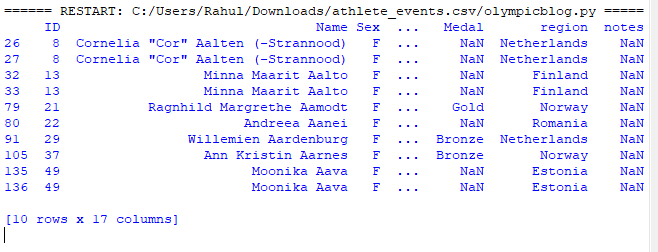### Top  5 countries who won the most medals

Here we are going to print the top 5 countries and show them in the graph with catplot.

## Python3

 `print``(goldMedals.region.value_counts().reset_index(name``=``'Medal'``).head())`   `totalGoldMedals ``=` `goldMedals.region.value_counts()` `.reset_index(name``=``'Medal'``).head(``5``)` `g ``=` `sns.catplot(x``=``"index"``, y``=``"Medal"``, data``=``totalGoldMedals,` `                ``height``=``6``, kind``=``"bar"``, palette``=``"muted"``)` `g.despine(left``=``True``)` `g.set_xlabels(``"Top 5 countries"``)` `g.set_ylabels(``"Number of Medals"``)` `plt.title(``'Medals per Country'``)` `plt.show()`

output: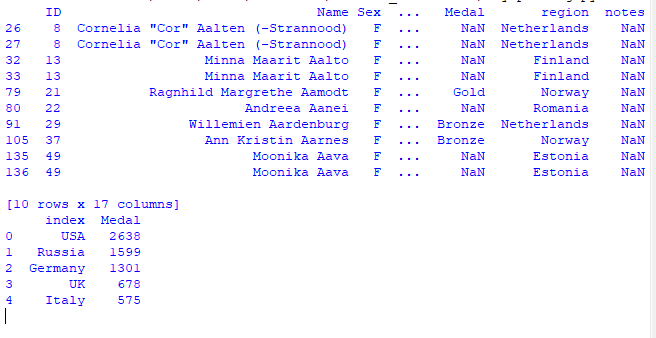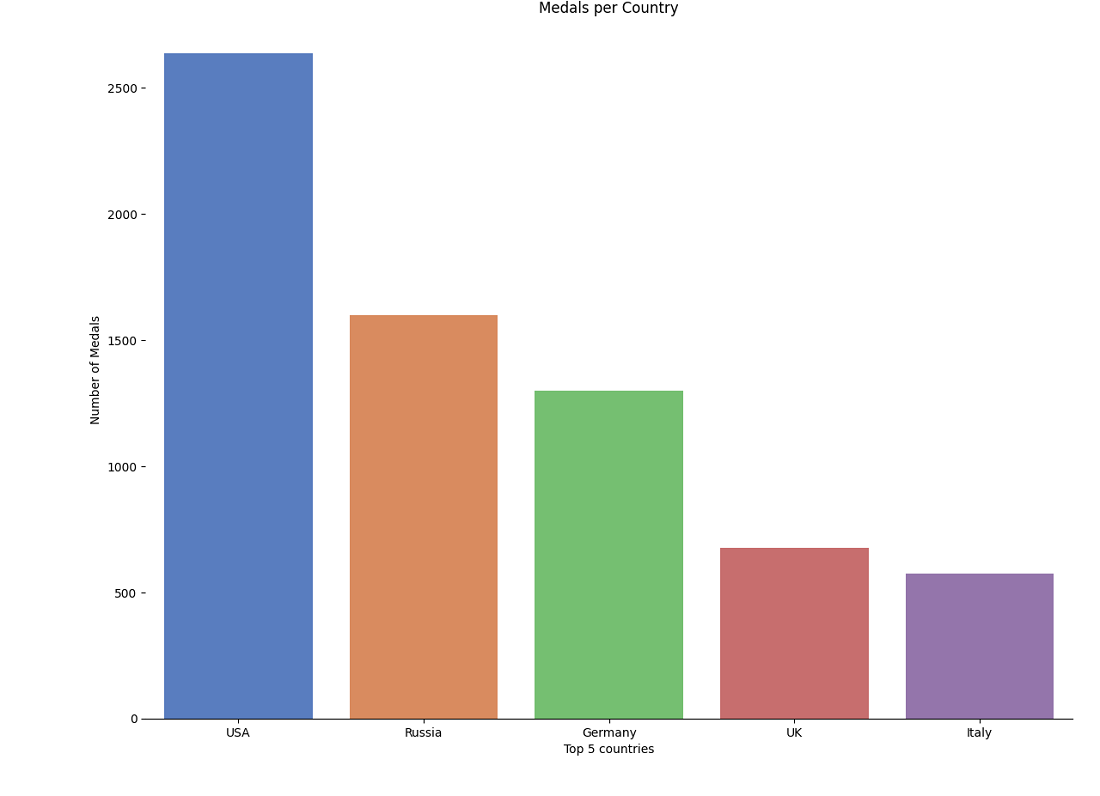### Players weight Analysis

Here we are going to see how weight over year for Male Lifters via graphical representation using pointplot.

## Python3

 `MenOverTime ``=` `merged[(merged.Sex ``=``=` `'M'``) &` `                     ``(merged.Season ``=``=` `'Summer'``)]` `wlMenOverTime ``=` `MenOverTime.loc[MenOverTime[``'Sport'``] ``=``=` `'Weightlifting'``]`   `plt.figure(figsize``=``(``20``, ``10``))` `sns.pointplot(``'Year'``, ``'Weight'``, data``=``wlMenOverTime, palette``=``'Set2'``)` `plt.title(``'Weight over year for Male Lifters'``)` `plt.show()`

Output :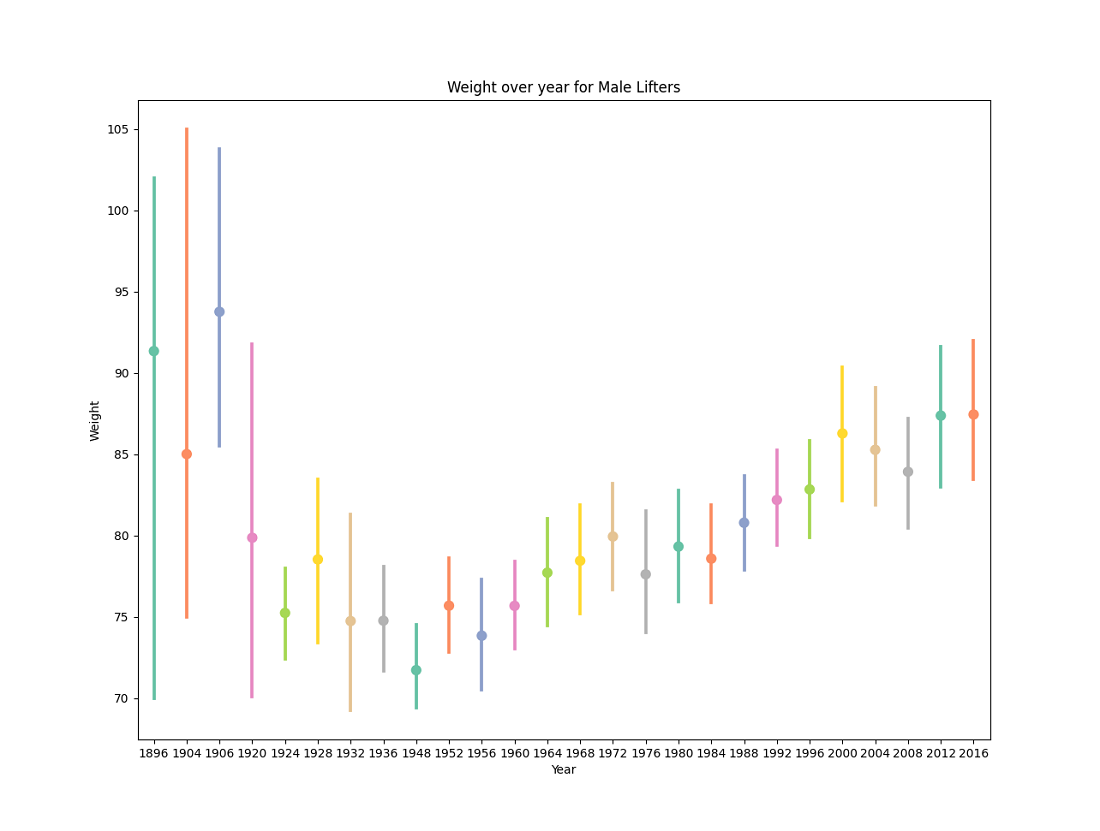My Personal Notes arrow_drop_up
Related Articles